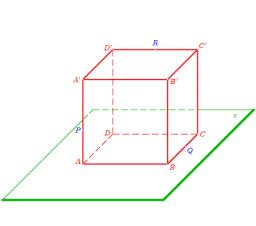# Calculate 6214

The cube A B C D A'B'C'D 'has a section area ACC'A' equal to 64 square root of 2 cm2 (square). Calculate the surface of the cube.

S =  384 cm2

### Step-by-step explanation:Did you find an error or inaccuracy? Feel free to write us. Thank you!

Tips to related online calculators
Pythagorean theorem is the base for the right triangle calculator.

#### You need to know the following knowledge to solve this word math problem:

We encourage you to watch this tutorial video on this math problem: